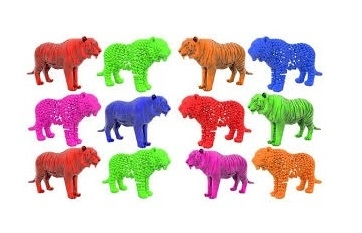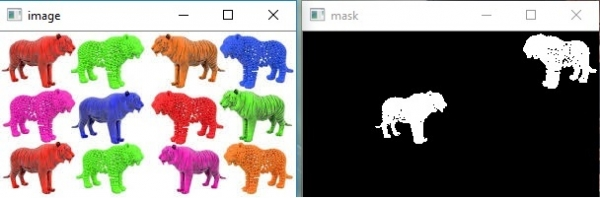# Detection of a specific color(blue here) using OpenCV with Python?

For many people, image processing may seem like a scary and daunting task but it is not as hard as many people thought it is. In this tutorial we’ll be doing basic color detection in openCv with python.

## How does color work on a computer?

We represent colors on a computers by color-space or color models which basically describes range of colors as tuples of numbers.

Instead of going for each color, we’ll discuss most common color-space we use .i.e. RGB(Red, Green, Blue) and HSV (Hue, Saturation, Value).

RGB basically describes color as a tuple of three components. Each component can take a value between 0 and 255, where the tuple (0, 0, 0) represents black and (255, 255, 255) represents white. For example, if we were to show a pure blue pixel on-screen, then the R value would be 0, the G value would be 0, and the B value would be 255.

Below are a few more examples of colors in RGB:

ColorRGB value
Red255, 0, 0
Orange255, 128, 0
Pink255, 153, 255

With HSV, a pixel is also represented by 3 parameters, but it is instead Hue, Saturation and Value. However, unlike RGB, HSV does not use the primary color to represent a pixel. Instead, it uses hue, which is the color or shade of the pixel.

The saturation is the intensity of the color, where a saturation of 0 represent 0 and a saturation of 255 is maximum intensity.Value  will tell how bright or dark the color is.

## Detecting the right color

So let’s first download the image with which we will be working with,Now that we have got the colors image, we can start the fun part. Just open your favourite python text editor or IDE and let’s get started.

import cv2
import numpy as np
import imutils
img = cv2.imread('color2.jpg')

In above line of code, first two lines handle all the imports. In third line, I’m importing imutils module, which helps in resizing images and finding the range of colors. In line 4 we’ve open the image.

hsv = cv2.cvtColor(img, cv2.COLOR_BGR2HSV)

Now we have convert the image to an hsv image because hsv helps to differentiate intensity from color.

lower_range = np.array([110,50,50])
upper_range = np.array([130,255,255])

Now we define the upper and lower limit of the blue we want to detect. To find these limit we can use the range-detector script in the imutils library. We put these values into a NumPy array.

mask = cv2.inRange(hsv, lower_range, upper_range)

Here we are actually creating a mask with the specified blue. The mask simply represent a specific part of the image. In this case, we are checking through the hsv image, and checking for colors that are between the lower-range and upper-range. The areas that match will an image set to the mask variable.

cv2.imshow('image', img)

while(True):
k = cv2.waitKey(5) & 0xFF
if k == 27:
break

cv2.destroyAllWindows()

Finally, we can show the original and mask image side by side to see the difference. If you want to understand what 0xFF means in the code read this. The code then waits for the user to hit the ‘Esc’ button which will quit it and destroy all the windows to cleanup.

## Final program

import cv2
import numpy as np
import imutils

hsv = cv2.cvtColor(img, cv2.COLOR_BGR2HSV)

lower_range = np.array([110,50,50])
upper_range = np.array([130,255,255])

cv2.imshow('image', img)

while(True):
k = cv2.waitKey(5) & 0xFF
if k == 27:
break

cv2.destroyAllWindows()

## OutputIn above, we see there are couples of black spots in the mask, that is the noise.

Updated on: 30-Jul-2019

2K+ Views